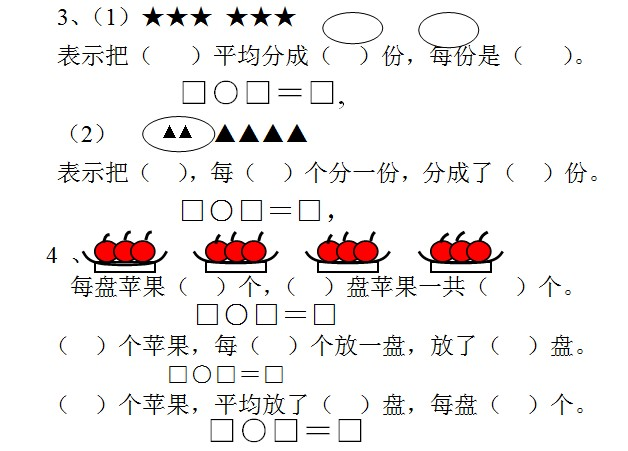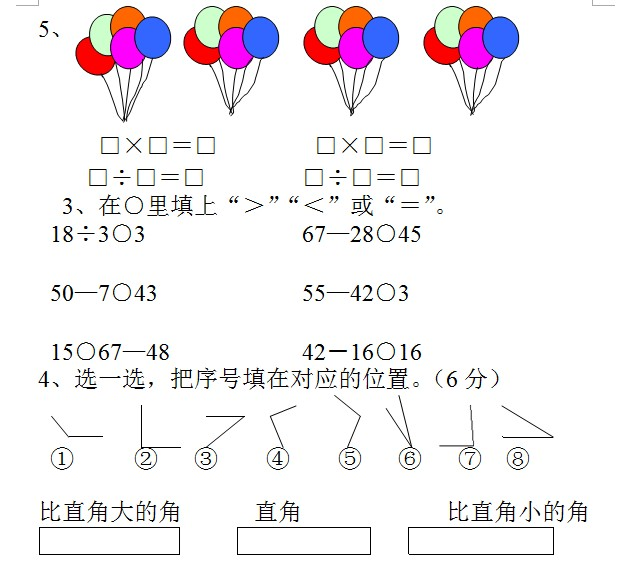## 小学二年级数学上册期中试卷 青岛版小学二年级上册数学期中测试题

青岛版小学二年级上册数学期中测试题

二年级                 姓名

一、你在3分钟内能完成吗？

6×3=   25+5=    30-9=    6×6=    2×6=

8×9=   5×6=    6×1=    3×3=    9×4=

16－4=  20＋5=   3×4=    0×8=    7×3=

30-6=   1×1=    5×7=    5×3=    5×9=

二、想想填填

1 、改写算式：

2+2+2+2+2                    或

6+6+6+6                       或

4+4+4+4+4+3                   或

2、4×7读作(        ),表示（  ）个（  ）相加或表示（  ）个（  ）相加。三、是非审判庭（对的在括号里打“√”，错的打“×”）。1、2×5读作2乘5。                     （  ）

2、2+2可以写成2×2，3+3可以写成3×3。 （  ）

3、6+6表示2个6相加。                 （  ）

4、3个7相加可以写成3×7。             （  ）

5、计算6×3和3×6可以用同一句口诀计算。（  ）

## 选择城市

#### 轻轻助手